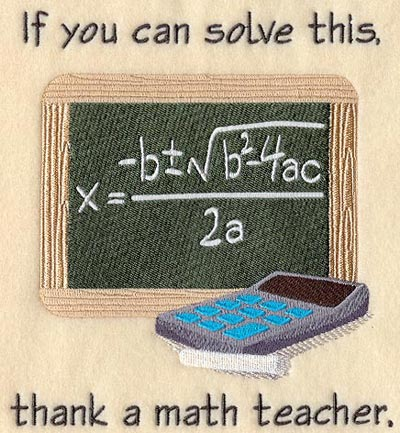Learn the 4 Things You Must Know Before Taking the GMAT!   Download it Now!

## Quadratic Equations, Simultaneous Equations, and InequalitiesWe go to great lengths at Dominate the GMAT to help you avoid doing difficult algebra on the GMAT. There are useful non-standard math techniques, for example, that can help you make variables your friend and turn otherwise problematic word problems into run-of-the-mill math problems.

Sometimes, however, solving algebra problems on the GMAT is simply unavoidable.

In this lesson you will specifically learn how to tackle three of the most commonly-tested “difficult” algebra concepts tested on the GMAT: Quadratic equations; Simultaneous equations; and Inequalities. But don’t worry — there won’t be a lot of long formulas to memorize (we still remember a song our 7th grade algebra teacher taught us to help us remember the Quadratic Formula, and don’t worry, we won’t be signing it to you here!); rather, we’ll focus on teaching you how the GMAT tests each of these topics and equip you with plenty of examples to illustrate everything you’ll need to master these GMAT algebra problem types.

Specifically, this GMAT video tutorial covers:

• Simultaneous equations, with multiple approaches and strategies so that you can choose the method that works best for you;
• GMAT quadratic equations, with an emphasis on the three most commonly-tested quadratic equations on the GMAT;
• The FOIL Method;
• How to factor quadratic equations, including the “reverse FOIL” method;
• Determining how many roots a quadratic equation has for determining sufficiency on GMAT Data Sufficiency questions;
• GMAT Inequalities;
• Numerous real GMAT practice problems with detailed answer explanations

### Cost: Only \$25!